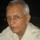Coffee Room
Discuss anything here - everything that you wish to discuss with fellow engineers.
12933 Members
Join this group to post and comment.Yes !! I do not need a calculator :)

I did not know that multiplying with 11 was this easy !
I came across a trick to multiply numbers with 11 and come up with your answer before the calculator .

For example : 32 * 11 [For numbers which add up to less than equal to 9]
Just add the two digits : 3+2=5 and place it in between the original number 32 and tada 352 is your answer 😀

99*11[Numbers which add up to a number greater than 9]
You again need to put this 18 in the middle of the original number but You have to add the 10s place number(obtained after addition) to the 1st digit of the original number .

77*11

Explanation : 7+7 = 14
Putting it in the middle : 7147
But you have to add 1 to 7 for the answer : (7+1)47 = 847

Start with this 2 digit number multiplication with 11 and display the MATHEMAGICIAN in you to your friends ! Enjoy 😀Bionic • Mar 11, 2014
56*11=(5+1)16=616..Bionic • Mar 11, 2014
67*11=(6+1)37=737...Bionic • Mar 11, 2014
34*11=(3(3+4)4)=374smorgasbord • Apr 18, 2014
you could do a lot more than that with Vedic maths47*11= (4+7)
=4117
=(4+1)17
=517 i got it"!!! 😀The Trachtenberg Speed System of Basic Mathematics book was available in the IIT Madras library in 1962. Later I bought it and went through all of it. I still use the techniques when applicable.

Multiplication by 11 (any number of digits) is done by a process called add the neighbour. What is shown above is a special case of this.